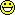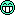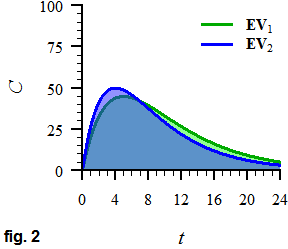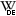Helmut
★★★Vienna, Austria,
2020-04-20 12:15
(363 d 16:52 ago)

Posting: # 21339
Views: 5,432

## [email protected]: COVID-19 ☣️ [Software]

Dear all,

since many of us are working at home now, consider donating your idle computation power to [email protected]* (see also theWiki-article). The software is available for Windows, macOS 10.7+, and 64bit Linux. With the end of March users provided 600,000 CPUs and 430,000 GPUs. Their distributed speed reached 1.3exaFLOPS last week, outperforming the currently leading supercomputer (IBM’sSummit) by one order of magnitude.Note that you have to own the machine. Otherwise, ask your company for permission.I lost 4,500 ranks since mid-March. Great because recently many users (including big players like Oracle, avast) joined the party (see the image).

The software allows a lot of adjustments. Since I don’t encode videos or play 3D-games, my GPU with its 64CUDA cores is bored. Hence, I fold on the GPU whilst leaving the CPU free for other stuff (like Phoenix, sim’s in). For a while I used six threads of the CPU but it’s not worth it – on the average out­performed by the GPU by a factor of ~8.5.

Don’t worry to toast your machine. The software monitors the CPU’s/GPU’s temperature and goes for at least 15 minutes into idle state if the system gets too hot. The software comes with a large database of CPUs/GPUs and ‘knows’ their critical temperatures. I never faced that in ½ year, neither on my workstation nor my notebook. The former runs 24/7 and is fanless.
If the noise of the fans in your machine annoys you, drag the slider in the Advanced Control to the left. You can pause/resume folding at any time. If you want to shut down your machine, I suggest to folding first. The project(s) will automatically resume folding after a reboot.

If you’re already running the software, update to v7.6.9 (released 2020-04-18) where you can prioritize COVID-19 projects:

Configure > Advanced > Cause Preference
Select

• Hosted at the Washington University in St. Louis, Center for Science and Engineering of Living Systems, Bowman lab.

Dif-tor heh smusma 🖖
Helmut SchützThe quality of responses received is directly proportional to the quality of the question asked. 🚮
Science Quotes
jag009
★★★

NJ,
2020-04-23 03:45
(361 d 01:22 ago)

@ Helmut
Posting: # 21343
Views: 3,751

## [email protected]: COVID-19 ☣️

Hi Helmut!

» The software allows a lot of adjustments. Since I don’t encode videos or play 3D-games, my GPU with its 64CUDA cores is bored. Hence, I fold on the GPU whilst leaving the CPU free for other stuff (like Phoenix, sim’s in R). For a while I used six threads of the CPU but it’s not worth it – on the average out­performed by the GPU by a factor of ~8.5.

Would my GPU help? Nvidia 2080ti...Helmut
★★★Vienna, Austria,
2020-04-23 07:34
(360 d 21:33 ago)

@ jag009
Posting: # 21344
Views: 3,751

## [email protected]: COVID-19 ☣️

Hi John,

» Would my GPU help? Nvidia 2080ti...Wow, definitely! Are you a gamer?

Dif-tor heh smusma 🖖
Helmut SchützThe quality of responses received is directly proportional to the quality of the question asked. 🚮
Science Quotes
jag009
★★★

NJ,
2020-04-24 05:51
(359 d 23:16 ago)

@ Helmut
Posting: # 21348
Views: 3,654

## [email protected]: COVID-19 ☣️

Hi Helmut!

» » Would my GPU help? Nvidia 2080ti...» Wow, definitely! Are you a gamer?

When I have free time. Yes... Will help out :)
Zoey
☆

NY,
2020-07-23 09:30
(269 d 19:37 ago)

@ Helmut
Posting: # 21781
Views: 1,641

## [email protected]: COVID-19 ☣️

I'm new here and this is a good find. I have a nephew who keeps talking about these folding projects which use multiple computers; will definitely share a link with him. He will be happy to be involved.
I came here looking to understand a few things about statistics (I have been on other forums too, as conversations really help). I have to write about the subject, some branches and their applications in real life, for a writer friend. I was planning to write a bit about Bioavailability, interpret z-score with examples of its application in these Covid times, and wrap up with geometric sequences in music. I will read and understand more, before I put it all together for him. This has been super helpful. I am hoping this writing assignment will grow to become a regular source of work. Thanks.
Helmut
★★★Vienna, Austria,
2020-07-23 14:02
(269 d 15:05 ago)

@ Zoey
Posting: # 21782
Views: 1,712

## BABE 101 (lenghty and spiced with PK)

Hi Zoey,

» I'm new here and this is a good find.

Welcome to the club.» I was planning to write a bit about Bioavailability,…Bioavailability (BA),bioequivalence (BE) and an excursion intopharmacokinetics (PK)…Absolute BA is a$$f=\frac{{\color{Blue}{AUC_{\textrm{EV}}}}\: {\color{Black}\times}\: {\color{Red}{D_{\textrm{IV}}}}}{\color{Red}{{AUC_{\textrm{IV}}}}\:{\color{Black}\times}\: {\color{Blue}{D_{\textrm{EV}}}}}\tag{1}$$where$$AUC=\int_{0}^{\infty}C(t)\tag{2}$$is theArea Under the Curve ($$\small{AUC}$$) as the integral of the concentration-time curve from the time of administration $$\small{(t=0)}$$ to infinite time. b $$\small{\textrm{EV}}$$ denotes an extravascular c and $$\small{\textrm{IV}}$$ an intravenous dose $$\small{D}$$. Sometimes you’ll find $$\small{F}$$ instead, which is simply $$\small{f}$$ in percent. In the example above $$\small{F=100\%}$$ because the same doses were administered and $$\small{AUCs}$$ are identical. BTW, $$\small{f_{\textrm{abs}}}$$ is an oxymoron.
If $$\small{\textrm{EV}}$$ is an orally administered solution and $$\small{f<1}$$, it means that the drug is not completely absorbed and/or partly degrades in the GI-tract and/or ismeta­bo­lized (already in thegut wall and/or the liver). From a clinical perspective a high $$\small{f}$$ is desirable because we can expect low variability. On the other hand, drugs with low $$\small{f}$$ can be effective as well. One example arebis­phos­pho­nates with $$\small{F\sim 2-4\%}$$.
The first part of the $$\small{\textrm{EV}}$$-curve showsabsorption, i.e., how the drugpermeates through the gastric membranes. That’s a continuous process which decreases with time because less drug remains in the GI-tract.
For some highly soluble and highly permeable drugs (e.g.,salbutamol) after an inhalation maximum concentrations are reached extremely fast and the onset of action occurs in less than 15 minutes. Good news for asthmatics.Relative BA compares two extravascular doses, or$$f_{\textrm{rel}}=\frac{{\color{Green}{AUC_{\textrm{EV}_1}}}\times {\color{Blue}{D_{\textrm{EV}_2}}}}{{\color{Blue}{AUC_{\textrm{EV}_2}}}\times {\color{Green}{D_{\textrm{EV}_1}}}}.\tag{3}$$If $$\small{\textrm{EV}_1}$$ is a pharmaceutical product and $$\small{\textrm{EV}_2}$$ an orally administered solution, it shows the influence of the formulation on BA. Here the $$\small{AUC\textrm{s}}$$ are identical but the maximum concentration ($$\small{C_{\textrm{max}}}$$) of $$\small{\textrm{EV}_1}$$ is lower and observed at a later time. That’s common since the formulation has to disintegrate first and then the drug dissolve.
If $$\small{\textrm{EV}_1}$$ is an immediate-release product it is desirable to match the solution as close as possible. If you have a toothache and take a pain-killer, you don’t want to get the effect after a couple of hours…
On the other hand, if $$\small{\textrm{EV}_2}$$ is an immediate-release product and $$\small{\textrm{EV}_1}$$ amodified-release product intended for chronic use, such a delayed and reduced $$\small{C_{\textrm{max}}}$$ is desirable. It might increase compliance (say, if the patient has to take the MR-product only once a day instead of the IR-product twice a day) and reduce adverse events due to extreme concentrations (after multiple doses the fluctuations of concentrations will be lower). In the late 1980s this was summarized in the catch-phrase “The flatter is better”.

In BE $$\small{\textrm{EV}_1}$$ is the new (Test, $$\small{\textrm{T}}$$) treatment and $$\small{\textrm{EV}_2}$$ the standard (Reference, $$\small{\textrm{R}}$$) treatment compared at the same dose. Hence, the dose cancels out from $$\small{(3)}$$ and we obtain$$f_{\textrm{rel}}=\frac{AUC_{\textrm{T}}}{AUC_{\textrm{R}}}.\tag{4}$$In fig. 2 above $$\small{f_{\textrm{rel}}=100\%}$$ but the maximum concentration of $$\small{\textrm{T}}$$ is lower than the one of $$\small{\textrm{R}}$$. Hence, regulatory agencies require not only a comparison of the “extent of absorption” $$\small{AUC}$$ but also of the “rate of absorption” (assessed by $$\small{C_{\textrm{max}}}$$ and – sometimes – its time $$\small{t_{\textrm{max}}}$$).
The definition as given in the Code of Federal Regulations, Title 21, Volume 5, Chapter I, Part 314, Suppart A, \$314.3:

Bioequivalence is the absence of a significant difference in the rate and extent to which the active ingredient or active moiety in pharmaceutical equivalents or pharmaceutical alternatives becomes available at the site of drug action when administered at the same molar dose under similar conditions in an appropriately designed study.

Since this a legal text, “significant” is used here in the common meaning (Merriam-Webster 2 a) and not in the statistical sense (M-W 2 b). The statistical methods are recommended in regulatory guidances. Since they are only a kind of “soft law”, one can deviate from them with a proper justification.
Also note the “site of action”. In general this relates toreceptors which may be located at a limited area (e.g., ones regulating blood pressure in thecarotid sinus), more widely spread (in the central nervous system), or almost ubiquitous (pain receptors throughout the body except the brain). Biopsies are definitely not an option and generally we measure concentrations in plasma.

Main assumptions and conditions in BE:
1. If products lead to similar concentrations in the systemic circulation, concentrations at the receptors are similar as well. In the strict sense that’s valid only in equilibrium (steady state after multiple doses), but due to thelaw of mass action already a single dose comes pretty close.
2. The subject population is selected with the aim of permitting detection of differences between products, i.e., the most sensitive condition (fasting or fed state, dose strength, regimen) is employed.
3. Based on I. and II., BE in healthy subjects is a surrogate for therapeutic equivalence (TE, i.e., studies in patients assessing safety and efficacy).
4. Some drugs (belonging to theBiopharmaceutics Classification System class I with high solubility and high permeability) behave like a solution – where BE is not required – and hence, demonstrating in vitro similarity is considered a valid surrogate for in vivo BE.
Generally we performinferential statistics, i.e., want to come to a conclusion with a certain probability. Thesignificance level of the test $$\small{\alpha}$$ is fixed by regulatory agencies with 0.05. That means that the chance that the new product is – erroneously – approved (although it is not bioequivalent) is ≤5%. This translates into alimited risk for patients: If concentrations would be too high, there might be problems with safety (adverse effects, toxicity) and if they would be too low, problems with (lacking) efficacy.
Although applied in the early days of BE, we abandoned testing for a significant difference decades ago. Instead a “clinically not relevant difference” $$\small{\Delta}$$ is used, which is generally 0.20 (or 20%). For Narrow Therapeutic Index Drugs (NTIDs) or Highly Variable Drugs (HVDs) $$\small{\Delta}$$ might be smaller or larger. d
Concentrations cannot be negative and therefore, assuming that they arenormally distributed$$C\in \mathbb{R}\:\vee \{-\infty,+\infty\}\tag{5}$$would be stupid. Instead we assume alognormal distribution$$C\in \mathbb{R}^+\:\vee \{>0,+\infty\}\tag{6}$$where concentrations are positive.
For convenience (our statistical models requireadditive effects) welog-transform our data. This affects also the limits of our acceptance range (AR) $$\small{\{\theta_1,\theta_2\}}$$, i.e., for the common $$\small{\Delta}$$ of 0.20 we obtain $$\small{\theta_1=1-\Delta=80\%}$$ and $$\small{\theta_2=1/(1-\Delta)=125\%}$$. Note that in the log-domain (where the analysis is performed) perfectly matching products with a $$\small{\textrm{T}/\textrm{R}}$$-ratio of 100% have $$\small{\log_{e} 1=0}$$ and limits are symmetric with $$\small{\log_{e} \theta_1\sim -0.2231}$$ and $$\small{\log_{e} \theta_2\sim +0.2231}$$.
Then we state two hypotheses:$$H_0:\; \mu_{\textrm{T}}/\mu_{\textrm{R}}\notin \{\theta_1,\theta_2\}\tag{7.1}$$$$H_{\textrm{a}}:\; \mu_{\textrm{T}}/\mu_{\textrm{R}}\in \{\theta_1,\theta_2\}\tag{7.2}$$where $$\small{H_0}$$ is the“Null Hypothesis” of bioinequivalence and $$\small{H_{\textrm{a}}}$$ the“Alternative Hypothesis” of bioequivalence. Note that we cannot “proove” anything in science; here we hope to reject the Null Hypothesis (and implicitly accepting the alternative of BE).
By means of Harry Potter’s magic wand we obtain from our study a“point estimate” (the most likely result) and its $$\small{100(1-2\alpha)=90\%}$$ econfidence interval (CI). Since sample sizes might be small (occasionally only twelve volunteers), we cannot use a test based on the normal distribution f but have to employ thet-distribution. There are three g possible outcomes (see also this presentation, slides 29–30):
1. The CI lies entirely within the acceptance range. We demonstrated that $$\small{\textrm{T}}$$ is bioequivalent to $$\small{\textrm{R}}$$ and open a bottle of champagne.
2. The CI overlaps the AR (i.e., at least one confidence limit is outside): The outcome is indecisive. It might be that $$\small{\textrm{T}}$$ is bioequivalent but we failed to demonstrate it. $$\small{\textrm{T}}$$ might have deviated more from $$\small{\textrm{R}}$$ than we assumed in study planning, and/or the variability might have been higher than assumed, and/or there might have been more dropouts than anticipated. h Whether it makes sense to repeat the study with more volunteers is a science in itself.
3. The CI lies entirely outside the AR. We demonstrated that $$\small{\textrm{T}}$$ is not bioequivalent to $$\small{\textrm{R}}$$. End of the story.
On another note, my friend Charlie DiLiberti once said:

Ask ten physicians how bioequivalence works and eleven of them will get it wrong.

Many believe [sic] that with an acceptance range of 80–125% two products might differ by 45% ignoring the fact that each of them had to pass BE with the confidence interval inclusion approach. i

The FDA retrospectively assessed 2,070ANDAs from 1996 to 2007. j It turned out that the average deviation from the RLD (reference-listed drug, i.e., the originator’s approved via anNDA) was 3.56% for $$\small{AUC}$$ and 4.35% for $$\small{C_{\textrm{max}}}$$. Furthermore, in nearly 98% of the BE studies […], the generic product’s $$\small{AUC}$$ differed from that of the innovator product by less than 10%…

Regrettably there is still a negative attitude against generic drugs amongst stakeholders (patients, pharmacists, physicians). k Possibly the perception of “cheaper = of poor quality” triggers anocebo effect in patients and physicians are mislead by various case reports about “failing drugs”.
In the early days of BE innovator companies tried hard tofalsify the concept of BE. They performed therapeutic equivalence (TE) studies in a large number of patients together with BE studies in healthy volunteers. The idea behind was: If the BE study passes and the TE study fails it would show that the concept is wrong. Lot of money spent, no avail; BE “works”. Soon the innovators realized that if they would succeed in falsifying the concept, they would shoot themselves in the foot. l They have also to demonstrate BE when scaling-up manufacturing from the small batches (likely less than 10,000 units) used in their Phase III studies to the final production batch size (sometimes millions of units). Furthermore, BE is also required if the formulation is substantially changed. People would be surprised how far the originator’s product might have ‘drifted’ from the original BA (see this post).

Hope that helps.

1. On the premise of linear pharmacokinetics, i.e., both absorption and elimination are independent from dose. THX to John for pointing that out!
We cannot administer drugs with low $$\small{f}$$ at the same dose because the $$\small{\textrm{IV}}$$ dose may lead to adverse effects. In a later stage of development dose-proportionality has to be assessed anyhow. Given recent advances in bioanalytics – which allows measuring extremely low concentrations – an alternative ismicrodosing.
2. Of course, we cannot measure the concentration until $$\small{t=\infty}$$. With afew exceptions (e.g., alcohol) – for $$\small{\textrm{EV}}$$ c once absorption is essentially complete – concentrations follow an exponential decrease, i.e.,$$C(t)=C_0 \exp (-k_{\textrm{el}}\times t)\tag{8}$$where $$\small{k_{\textrm{el}}}$$ is the elimination rate constant.In practice we measure as long as we can (in the example for 24 hours), estimate $$\small{k_{\textrm{el}}}$$ bylog-linear regression and perform an extrapolation by$$AUC_{0-\infty}=\int_{0}^{t}C(t)+\frac{\widehat{C_{{\textrm{t}}}}}{k_{\textrm{el}}}\tag{9}$$BTW, have you ever heard the term “half life”?
It’s obtained by $$\small{t_{1/2}=-\log_{e} 2 / \small{k_{\textrm{el}}}}$$. In my examples I had $$\small{k_{\textrm{el}}}\;0.1733$$ and therefore, $$\small{t_{1/2}=0.6931/0.1733\sim 4\;\textrm{hours}.}$$
To the right a plot like fig. 1 but with concentrations in a logarithmic scale. After the $$\small{\textrm{IV}}$$ dose every $$\small{t_{1/2}}$$ the concentration drops by 50%:
$$\small{100 \xrightarrow[4\,\textrm{h}:\,^1/_2]{t_{1/2}}50 \xrightarrow[8\,\textrm{h}:\,^1/_4]{t_{1/2}}25 \xrightarrow[12\,\textrm{h}:\,^1/_8]{t_{1/2}}12.5 \xrightarrow[16\,\textrm{h}:\,^1/_{16}]{t_{1/2}}6.25 \xrightarrow[20\,\textrm{h}:\,^1/_{32}]{t_{1/2}}3.125 \xrightarrow[24\,\textrm{h}:\,^1/_{64}]{t_{1/2}}1.5625.}$$
After five half lives (here 20 hours) already 96.875% of the drug left the systemic circulation. We see also that after $$\small{2\times t_{{\textrm{max}}}}$$ (8 hours) of the $$\small{\textrm{EV}}$$ dose its elimination runs in parallel to the one of the $$\small{\textrm{IV}}$$ dose, meaning that absorption is essentially complete. That’s good because we can estimate its $$\small{k_{\textrm{el}}}$$ which we need in extrapolating the $$\small{AUC}$$ acc. to $$\small{(9)}$$.
3.Extravascular means any route of administration which is not intravenous (a fast injection or an infusion), e.g., oral, sublingual, rectal, vaginal, topical (transdermal systems, creams, ointments, …), inhalation, intranasal, ophthalmic, intramuscular and subcutaneous injections, …
Hence, it can be more complicated than in the simple examples before.
In fig. 4 $$\small{\textrm{EV}_1}$$ is a so-called “pulsatile” oral formulation, which releases 60% of the dose fast (for a rapid onset of effect) and 40% with a slower absorption $$\small{t_{1/2}}$$ after four hours (for maintenance of effect). $$\small{\textrm{EV}_2}$$ is atransdermal system, where the patch is removed after eight hours.
4. For NTIDs in some jurisdictions $$\small{\Delta}$$ is fixed at 0.10, which gives an acceptance range (AR) of 90.00–111.11%. The FDA recommends that the AR is “scaled” based of the variability of the reference-drug and – in rare cases – it might be even narrower.
For HVDs $$\small{\Delta}$$ some jurisdictions recommend a fixed $$\small{\Delta}$$ of 0.25, which gives an AR of 75.00–133.33%. Others recommend “reference-scaling”, where $$\small{\Delta\leqslant 0.3016}$$ might lead to an AR of up to 69.84–143.19%. HVDs are safe drugs because they have a flatdose-response curve (large differences in concentrations lead to small differences in effects). If they would have a step dose-response curve they would not get approved first because in the Phase III studies – due to the high variability – there would be an unacceptable high number of patients experiencing AEs (toxicity) and lacking effects. Furthermore, if there would problems become evident in Phase IV studies (post marketing) orpharmacovigilance they would have been taken off the market. In short, HVDs “work” despite their high variability which justifies a wider AR for them.
5. Why not the 95% CI which is employed inPhase III clinical studies of a new treatment? In those studies aone-sided test is performed (hoping that the treatment is superior to placebo or an established standard treatment). Hence, the patient’s risk (the Type I Error) is 5%. Yes, there is a 5% chance that even a “blockbuster-drug” does not perform better than snake-oil… In BE we want limit the patient’s risk to 5% as well. However, in a particular patient concentrations can be either too low or too high but obviously not at the same time. Hence, the 90% CI covers both scenarios in the population of patients.
6. Like in your example about $$\small{Z}$$-scores. With 50 students it’s fine because the t-distribution approaches the normal distribution reasonably fast. Most people happily apply $$\small{\mathcal{N}}$$ if $$\small{n\geqslant 30}$$. However, in BE we are a cautious bunch and always use tests based on the t-distribution.
7. From a regulatory point of view the outcome is dichotomous; either the study passed (#1) or it failed (#2 or #3). From the producer’s perspective only #3 is a disaster. In the gray zone (#2) there is still hope.
8. All applicable regulatory guidances mandate that the study is designed for a certain – high –power (i.e., 80–90%). Quoting the guideline “Statistical Principles for Clinical Trials” of the International Council for Harmonisation:
• The number of subjects in a clinical trial should always be large enough to provide a reliable answer to the questions addressed.
1 – power is theType II Error or the “producer’s risk” that the study fails to demonstrate BE although the products are BE. Including more volunteers in order to decrease the chance of failing (e.g., below 10%) likely will not be accepted by the ethics committee / the institutional review board.
9. If the true $$\small{\textrm{T}/\textrm{R}}$$-ratio would be 80% or 125%, even a study with the entire population of our lovely planet will fail to show BE since the lower (or upper) confidence limit would be outside the AR.
10. Davit BM, Nwakama PE, Buehler GJ, Conner DP, Haidar SH, Patel DT, Yang Y, Yu LX, Woodcock J. Comparing Generic and Innovator Drugs: A Review of 12 Years of Bioequivalence Data from the United States Food and Drug Administration. Ann Pharmacother. 2009; 43(10): 1583–97. doi:10.1345/aph.1M141. PMID 19776300.
11. Dunne SS, Dunne CP. What do people really think of generic medicines? A systematic review and critical appraisal of literature on stakeholder perceptions of generic drugs. BMC Medicine. 2015; 13:173. doi:10.1186/s12916-015-0415-3. PMID 26224091.Open access.
12. The Phase III study of the firststatin (simvastatin) was performed in thousands (‼) of patients. Such a high number was required to demonstrate superiority to a low-cholesterol diet; the study took 5½ years. Can you imagine how many patients would be required to show a difference between two products of ≤20%? Maybe 12,000 and the study would take ages to complete.
BTW, Phase III studies of two COVID-19 vaccine candidates started in 30,000 volunteers each…

Dif-tor heh smusma 🖖
Helmut SchützThe quality of responses received is directly proportional to the quality of the question asked. 🚮
Science Quotes
Zoey
☆

NY,
2020-07-24 10:10
(268 d 18:57 ago)

@ Helmut
Posting: # 21784
Views: 1,561

## Thank you

» Hope that helps.

Yes, some of it makes sense Helmut. I am not trained to understand it all. Many thanks.
Helmut
★★★Vienna, Austria,
2020-07-24 12:33
(268 d 16:34 ago)

@ Zoey
Posting: # 21786
Views: 1,508

## Welcome

Hi Zoey,

» I am not trained to understand it all.

No worries. If you have questions, feel free to ask.

» Many thanks.

Welcome.

Dif-tor heh smusma 🖖
Helmut SchützThe quality of responses received is directly proportional to the quality of the question asked. 🚮
Science Quotes
Admin contact
21,419 posts in 4,475 threads, 1,510 registered users;
online 12 (0 registered, 12 guests [including 4 identified bots]).
Forum time: Monday 05:08 UTC (Europe/Vienna)

There is one certainty in drug development
and statistics that one can depend on:
the data are always late.    Scott Patterson and Byron Jones

The Bioequivalence and Bioavailability Forum is hosted byIng. Helmut Schütz